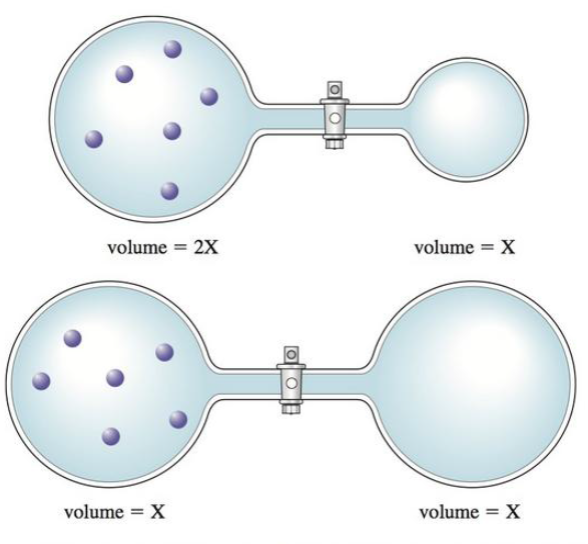# Consider the flasks in the following diagrams. Assuming the connecting tube has negligible volume, draw what each diagram will look like after the stopcock between the two flasks is opened. Also, solve for the final pressure in each case, in terms of the original pressure. Assume temperature is constant.### Chemistry: An Atoms First Approach

2nd Edition
Steven S. Zumdahl + 1 other
Publisher: Cengage Learning
ISBN: 9781305079243

#### Solutions

Chapter
Section### Chemistry: An Atoms First Approach

2nd Edition
Steven S. Zumdahl + 1 other
Publisher: Cengage Learning
ISBN: 9781305079243
Chapter 8, Problem 28Q
Textbook Problem
677 views

## Consider the flasks in the following diagrams.Assuming the connecting tube has negligible volume, draw what each diagram will look like after the stopcock between the two flasks is opened. Also, solve for the final pressure in each case, in terms of the original pressure. Assume temperature is constant.

Interpretation Introduction

Interpretation: The final diagram and the final pressure in each case have to be given.

Concept Introduction:

Boyle’s law: For a given mass of a gas at constant temperature, the pressure (P) is inversely proportional to its volume (V). The Boyle’s law can be expressed as,

P=(nRT)1V

Where nRT=constant

It can also be given as,

1VPV=constant

### Explanation of Solution

Explanation

Determine the final pressure and draw the diagram for first case.

In the first diagram, the flask 2X volume must have four gas particles and X volume flask must have two gas particles.

By Boyle’s law, the pressure in the two flasks after stopcock is opened is

P1V1=P2V2P2=P1V1V2=P1×2X3X=23P1

The first diagram will have four particles in gas in the 2X volume flask and X volume flask will have two gas particles. The total volume of flask will be 3X after stopcock is opened.

Figure 1:

Over the entire volume ( 3X ) the six total gas particles will be evenly scattered

### Still sussing out bartleby?

Check out a sample textbook solution.

See a sample solution

#### The Solution to Your Study Problems

Bartleby provides explanations to thousands of textbook problems written by our experts, many with advanced degrees!

Get Started

Find more solutions based on key concepts
The Dietary Reference Intakes (DRI) were devised for which of the following purposes? a. to set nutrient goals ...

Nutrition: Concepts and Controversies - Standalone book (MindTap Course List)

• use bind theory to describe bonding in solids.

Chemistry for Engineering Students

Match the concepts:

Human Biology (MindTap Course List)

In what way has biotechnology had an impact on agriculture in the United States?

Human Heredity: Principles and Issues (MindTap Course List)

24. Name each compound:

Chemistry In Focus

Review. A small object with mass 4.00 kg moves counterclockwise with constant angular speed 1.50 rad/s in a cir...

Physics for Scientists and Engineers, Technology Update (No access codes included)

What is pollution? What factors determine how dangerous a pollutant is?

Oceanography: An Invitation To Marine Science, Loose-leaf Versin